Mobile QR Code1. (Dept. of Electrical Engineering, Pusan National University, Busan, Korea)
2. (Samsung Electronics, Gyeonggi, Korea)

CMOS, millimeter-wave, mixer, 5G applications

## I. INTRODUCTION

In recent years, new-generation wireless communi-cation standard such as 5G cellular standard is being developed to provide Gb/s data transfers in the wireless data traffic (1-3). As broadband spectrum can be used to support Gb/s data transfers, many countries such as the US, EU, Korea, China, and Japan have planned to use the millimeter-wave (mmWave) band for 5G communication services. The 28-GHz band is a promising candidate among the 5G mmWave frequency bands in Korea. A direct-conversion receiver architecture can be used to implement a low-power 5G mmWave transceiver for energy efficiency (4). The linearity of the direct-conversion receiver front-end depends on the down-conversion mixer. Therefore, it is important to implement the highly linear down-conversion mixer with low power consumption. In this letter, a highly linear low-power 28-GHz mmWave down-conversion mixer employing a transformer-based topology and a derivative superposition linearization technique with low-magnetic-coupled source degeneration inductors is proposed to improve the linearity degraded by magnetically coupled distortion. It is implemented and demonstrated using a 65-nm CMOS process.

## II. PROPOSED CIRCUIT DESIGN

Fig. 1 shows the simplified schematic of the proposed mmWave down-conversion mixer with a local oscillator (LO) buffer employing the derivative superposition technique for a direct-conversion receiver. The RF input frequency range is from 26.5 to 29.5 GHz, and the output signal has a 3-dB bandwidth of 1 GHz. At the initial stage of the schematic circuit design, the modelled element or S-parameter of a stand-alone inductor is used as the source degeneration inductor of the main (M$_{1}$) and auxiliary (M$_{2}$) transistor without considering the coupling factor. In this case, the linearity performance of the down-conversion mixer is optimized without including the magnetic coupling effect between two source degeneration inductors, L$_{\mathrm{S1}}$ and L$_{\mathrm{S2}}$. The inductances of the optimized L$_{\mathrm{S1}}$ and of L$_{\mathrm{S2}}$ are 340 pH and 850 pH at 28 GHz, respectively. All line widths and spaces of the two inductors (L$_{\mathrm{S1}}$ and L$_{\mathrm{S2}}$) are 2 ${\mathrm{\mu}}$m. The quality factor of L$_{\mathrm{S1}}$ and of L$_{\mathrm{S2}}$ is 14 and 17 at 28 GHz, respectively. However, as two source degeneration inductors are conventionally placed facing two vertexes or two parallel lines as shown in Fig. 2(a), the adjacent layout arrangement of the source degeneration inductors causes two inductors to couple magnetically. Fig. 3(a) shows the coupling coefficient, k versus the distance between the conventional source degeneration inductors of Fig. 2(a). Even though the coupling coefficient, k between the source degeneration inductors is small, the linearity performance is not improved at the post-layout simulation including the electromagnetic effect because the coupling factor changes the optimized design point. When the electromagnetic effect is included in our design, the coupling coefficient k that is less than 0.05 does not degrade the linearity performance of the down-conversion mixer without considering the coupling factor between two source degeneration inductors. As shown in Fig. 3(a), two source degeneration inductors are separated by more than 400 ${\mathrm{\mu}}$m for k of 0.05. However, the parasitic inductance by the additional routing line is induced and more silicon area by separation is occupied. Therefore, compact and low-magnetic-coupled source degeneration inductors are required to maintain the optimized design parameters of the down-conversion mixer without considering the magnetic coupling factor between the source degeneration inductors.

Fig. 1. Simplified schematic of the down-conversion mixer with LO buffer using the proposed low-magnetic-coupled source degeneration inductors.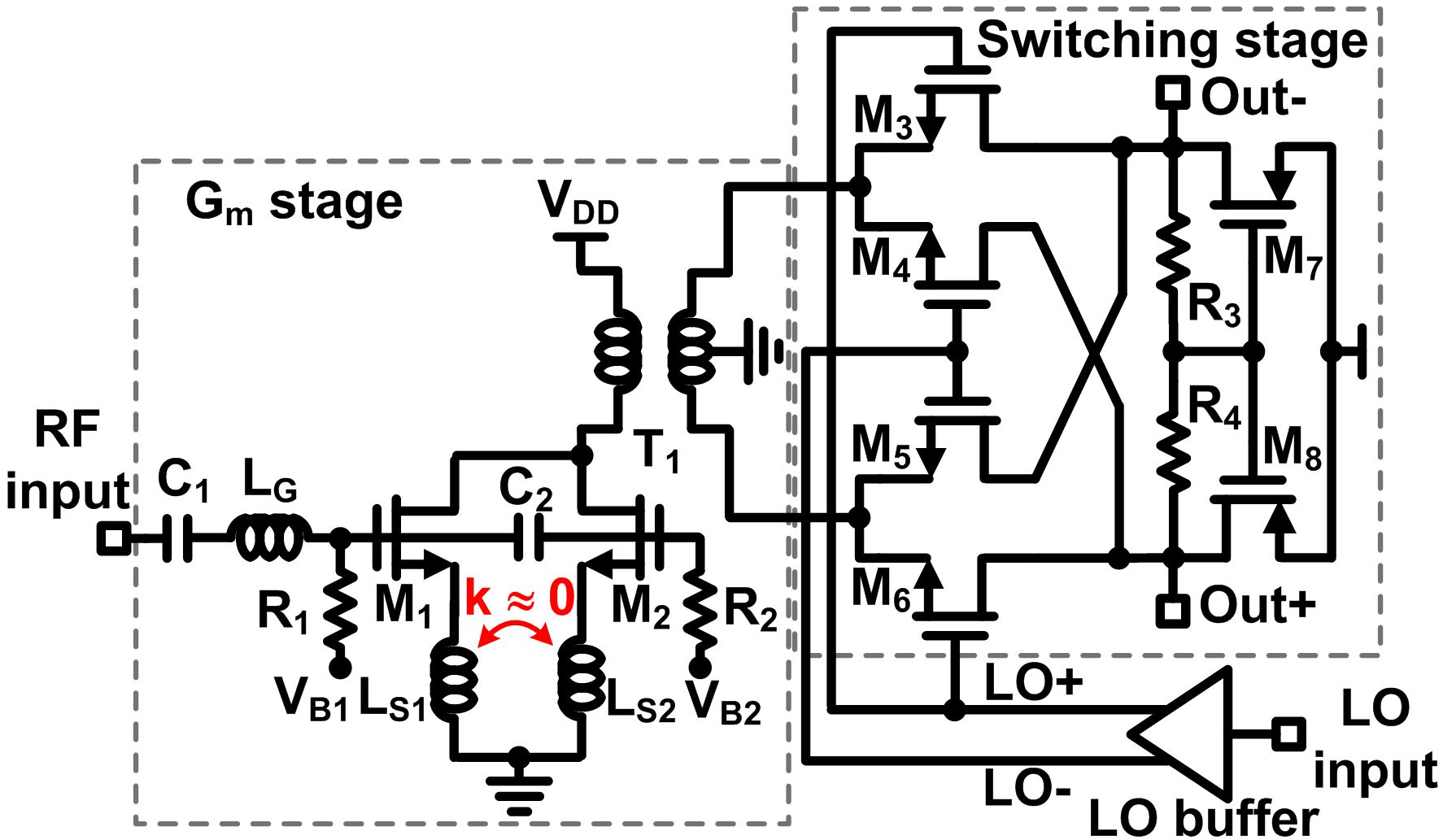Fig. 2. Source degeneration inductor configuration (a) Conventional source degeneration inductors, (b) Proposed source degeneration inductors.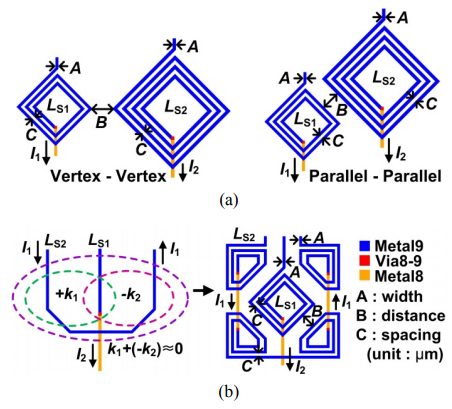Fig. 3. Simulated coupling coefficient k (a) k versus distance between two conventional inductors at 28 GHz, (b) Comparison of k with respect to frequency.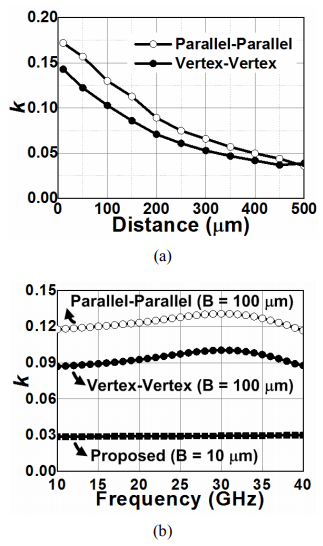As shown in Fig. 2(b), the magnetic flux value on the left side between the U-shaped line inductor and the straight line inductor is opposite to that of the magnetic flux on the right side between the U-shaped line and the straight line. Therefore, the total coupling factor between two inductors can be zero. By applying this concept, the low-magnetic-coupled source degeneration inductors have been proposed, as shown in Fig. 2(b). The inductance values of L$_{\mathrm{S1}}$ and L$_{\mathrm{S2}}$ used at transistors M$_{1}$ and M$_{2}$ are equal to those of the inductors without the coupling factor used at the optimized stage of our circuit design. The quality factor of the proposed inductors L$_{\mathrm{S1}}$ and L$_{\mathrm{S2}}$ are 13 and 12.5 at 28 GHz, respectively. As shown in Fig. 3(b), the coupling coefficient k between the proposed inductors is approximately 0.03 in the frequency range from 10 GHz to 40 GHz. The simulated linearity performance of the down-conversion mixer using this proposed source degeneration inductors is the same as that of the down-conversion mixer using stand-alone L$_{\mathrm{S1}}$ of 340 pH and L$_{\mathrm{S2}}$ of 850 pH.

The bias voltages (V$_{\mathrm{B1}}$ and V$_{\mathrm{B2}}$) of the main and auxiliary transistors are supplied from the current mirror bias circuits (5). To avoid stacking for high linearity at a low supply voltage, the G$_{\mathrm{m}}$ stage and switching stage are coupled magnetically using on-chip 2:1 transformer T$_{1}$. The impedance between the output of the G$_{\mathrm{m}}$ stage and the input of the switching stage is matched by the on-chip 2:1 transformer. The switching pairs (M$_{3}$${-}$M$_{6}$) are driven by the single-to-differential LO buffer, which comprises a two-stage common-source amplifier with an on-chip balun transformer consuming 10 mA from a 1-V supply voltage. The proposed mixer with LO buffer was designed using the extracted S-parameters of all passive components such as capacitors, inductors, transformers, routing lines, supply and ground plates through an electromagnetic simulator.

## III. EXPERIMENTAL RESULTS

Fig. 4. Chip photograph of the proposed mmWave down-conversion mixer with LO buffer.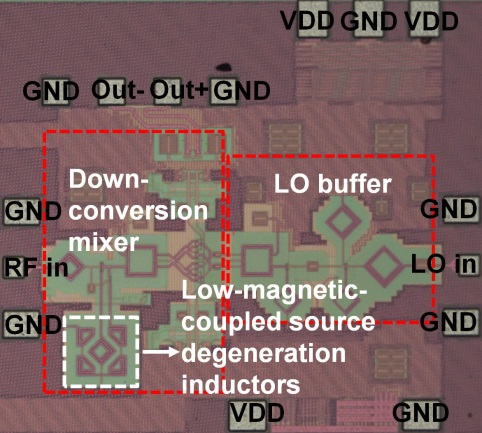Fig. 5. Measured results of the down-conversion mixer with LO buffer (a) Measured return losses of RF and LO input, (b) Measured conversion gain, OIP3 and NF versus LO input power, where RF frequency is 28 GHz, LO frequency is 16~GHz, two-tone spacing frequency is 20 MHz, respectively.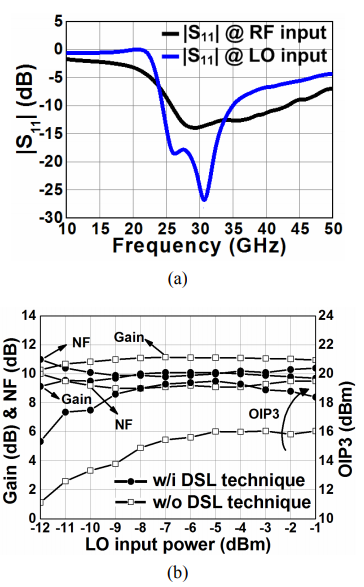The proposed mixer with LO buffer was implemented using a 65-nm CMOS process. The chip photograph of the proposed mixer with LO buffer is shown in Fig. 4. The core areas of the mixer and LO buffer are 350 ${\mathrm{\mu}}$m ${\times}$ 500 ${\mathrm{\mu}}$m and 360 ${\mathrm{\mu}}$m ${\times}$ 300 ${\mathrm{\mu}}$m, respectively. The current consumption of the mixer and LO buffer is 11 mA and 10 mA from a 1-V supply voltage, respectively. Fig. 5(a) shows the measured return losses of the RF input and LO input. The measured input matching $\left(\left|\mathbf{S}_{11}\right|\right)$ of the RF input and LO input is below -10 dB at the operating frequencies, respectively. Fig. 5(b) presents the measured conversion gain, NF, and third-order output intercept point (OIP3) versus the LO input power of the down-conversion mixer with and without the derivative superposition linearization (DSL) technique.

The derivative superposition method ensures approximately a 4-dB OIP3 improvement at -7 dBm of the LO input power without any additional power consumption. Table 1 summarizes the measured performances of the proposed down-conversion mixer with LO buffer and the recently published mmWave down-conversion mixers. The proposed mixer has excellent figure of merit compared with the recently published mmWave mixers.

Table 1. Performance comparison with other mmWave down-conversion mixers

    This work Operating frequency (GHz) 57-66 31 57-66 26.5-29.5 Gain (dB) 12 3.4 >5.6 10.1 OIP3 (dBm) -0.5 21.4 >12.4 19.3 NF (dB) < 15 9.5 <11 9.9 LO power (dBm) -13 3 -10* -7* Power consumption (mW) 8..8 @ 1.2V (Mixer+IF Buffer + LO buffer) 21.2 @ 1.5V (Only mixer) 10 / 11* @ 1V (Mixer + IF balun + LO buffer) 11 / 21* @ 1V (Mixer + LO buffer) Technology 90 nm CMOS 45 nm SOI CMOS 65 nm CMOS 65 nm CMOS Area(mm$^2$) 0.24 0.8 0.14 / 0.22* 0.18 / 0.28* F.O.M(1)  0.41 @ 60 GHz 25.5 @ 31 GHz 20.1 / 10* @ 60 GHz 24.7 / 12.9* @ 28 GHz

* value with LO buffer,

##### (1)
$^{\mathrm{F.O.M.=\frac{Gain[abs]\cdot IIP3[mW]}{P_{DC}[mW]}\cdot \frac{1}{(NF- 1)[abs]}\cdot f[GHz]}}$

## IV. CONCLUSIONS

A 28-GHz down-conversion mixer with low-coupling source degeneration inductors was proposed for 5G mmWave applications. As the proposed mixer has excellent performance compared with the previously published ones, this mixer could be suitable for 5G mmWave cellular receivers.

### ACKNOWLEDGMENTS

This research was supported by Samsung Electronics. This research was supported by Basic Science Research Program through the National Research Foundation of Korea funded by the Ministry of Education (NRF-2016R1D1A1A09919228) and the Ministry of Science and ICT, Korea, under the Information Technology Research Center support program (IITP-2019-2017-0-01635) supervised by the Institute for Information & communications Technology Promotion.

### REFERENCES

1
Hossain E., Hasan M., Mar 2015, 5G cellular: key enabling technologies and research challenges, IEEE Trans. Instrum. Meas., Vol. 18, No. 3, pp. 11-212
Byeon C. W., Park C. S., Jun 2017, A high dara rate, high output power 60 GHz OOK modulator in 90 nm CMOS, Journal of Semiconductor Technology and Science, Vol. 17, No. 3, pp. 341-3463
Shin B., Kim C., Lee O., Park S.R., Noh S., Nam I., Aug 2018, A 24 GHz receiver front-end with improved I/Q balanced LO generator in 65-nm CMOS for surveillance radar systems, Journal of Semiconductor Technology and Science, Vol. 18, No. 4, pp. 475-4794
Kim H. T., Park B.S., Song S.S., Moon T.S., Kim S.H., Kim J.M., Chang J.Y., Ho Y.C., 2018, A 28-GHz CMOS direct conversion transceiver with packaged 2 $\times$ 4 antenna array for 5G cellular system, IEEE J. of Solid-State Circuits, Vol. 53, No. 5, pp. 1245-12595
Lim Y.W., Nam I., Kim H.T., Lee K., 2009, A highly linear wideband up-conversion differential CMOS micromixer using IMD3 cancellation for a digital TV tuner IC, IEEE Microw. Wireless Compon. Lett., Vol. 19, No. 2, pp. 89-916
Byeon C. W., Lee J. J., Song I. S., Park C. S., Mar 2012, A 60 GHz current-reuse LO-boosting mixer in 90 nm, CMOS, IEEE Microw. Wireless Compon. Lett., Vol. 22, No. 3, pp. 135-1377
Wu C.-L., Yu C., O K. K., 2015, Amplification of nonlinearity in multiple gate transistor millimetre wave mixer for improvement of linearity and noise figure, IEEE Microw. Wireless Compon. Lett., Vol. 25, No. 5, pp. 310-3128
Choi C., Son J. H., Lee O., Nam I., Mar 2017, A +12-dBm OIP3 60-GHz RF downconversion mixer with an output-matching, noise- and distortion-canceling active balun for 5G applications, IEEE Microw. Wireless Compon. Lett., Vol. 27, No. 3, pp. 284-286## Author

Changyeol Kim received the B.S. degree in electronic and electrical engineering from Pusan National University, Busan, Korea, in 2016, the M.S. degree in electrical and computer engineering at Pusan National University, Busan, Korea, in 2019.

He is currently working at Silicon Works since 2019.

Minsu Kim received his B.S. and M.S degrees in electronic and electrical engineering from Pusan National University, Busan, Korea, in 2013 and 2015, respectively.

Since 2015 he is research engineer in LIG Nex1.

His research interests include Satellite Communication System.

Yangji Jeon received the B.S. degree in electronic engineering from Pukyong National University, Busan, Korea, in 2017 and is currently working toward the M.S. degree in electrical engineering at Pusan National University, Busan, Korea.

Her main interests are CMOS RF/mmWave/analog circuits for wireless communications.

Ockgoo Lee received the B.S. degree in electrical engineering from Sungkyunkwan University, Korea, in 2001, the M.S. degree in electrical engineering from the KAIST, Korea, in 2005, and the Ph.D. degree in electrical and computer engineering from the Georgia Institute of Technology, USA, in 2009.

Upon completion of the doctoral degree, he joined Qualcomm Inc., USA, as a Senior Engineer, where he was involved in the development of transmitters and integrated passive circuits on mobile applications.

He is currently a faculty member with the Department of Electrical Engineering, Pusan National University, Korea.

His research interests include high-frequency integrated circuits and system design for wireless communications and biomedical applications.

Ilku Nam received the B.S. degree in EE from Yonsei University, Korea, in 1999, and the M.S. and Ph.D. degrees in EECS from the KAIST, Korea, in 2001 and 2005, respec-tively.

From 2005 to 2007, he was a Senior Engineer with Samsung Electronics, Gyeonggi, Korea, where he was involved in the development of mobile digital TV tuner IC.

In 2007, he joined the School of Electrical Engineering, Pusan National University, Busan, Korea, and is now a Professor.